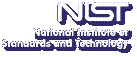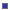Up# Most Accurate Molecular Quantum Computations to Date Performed

## January 2006

Computational scientists at NIST and Indiana University have achieved record levels of accuracy in the development of computational methods for the virtual measurement of fundamental properties of molecules. Their recent result for the ground state of dihydrogen (H2) represents the highest level of accuracy ever reached (10-12 hartree) in molecular quantum computations (except for trivial one-electron cases). H2 has the distinction of being the first molecule whose dissociation energy was correctly predicted by quantum mechanical calculation (1968) before being measured reliably by experiment. Today we may be witnessing again a situation in which quantum mechanical calculations yield more accurate determinations of this fundamental property than can be measured experimentally.

Exact analytical solutions to the nonrelativistic molecular electronic Schrodinger equation are known (in the Born-Oppenheimer approximation) only for the one-electron H2+ ion and other equivalent one electron systems. However, very high precision approximations are now available for molecular hydrogen (a two-electron system). MCSD computational scientist James Sims, in collaboration with Stanley Hagstrom of Indiana University, has calculated Born-Oppenheimer energies of 1Σg+ states of H2 using up to 7034 expansion terms in confocal elliptical coordinates with explicit inclusion of interelectronic distance coordinates up through r127. Sims and Hagstrom calculate Born-Oppenheimer (BO) energies for various internuclear distances in the range of 0.4 to 6.0 bohr with an error of 1 in the 13th digit. For example, the nonrelativistic energy is -1.1744 7571 4220(1) hartree at R = 1.4 bohr, and is -1.1744 7593 1399(1) hartree at the equilibrium R = 1.4011 bohr distance. In each case Sims and Hagstrom obtained lower (i.e., more accurate) energies than previously reported results. In an article recently accepted by the Journal of Chemical Physics, Sims and Hagstrom discuss how these best calculations to date on the ground state of neutral hydrogen were accomplished.

Almost all results reported in their paper were obtained using quadruple precision (approximately 30+ digit) floating point subroutines written in Fortran 90. In addition, multiple precision floating point arithmetic was used for the Rudenberg f function, on which all integrals depend, and which is subject to differencing problems. To address these differencing problems the authors systematically increased the number of decimal digits used for the f part of the calculation up to a maximum of 160 decimal digits. Parallel processing proved essential for obtaining results over a range of R from 0.4 to 6.0 bohr. The authors solved the secular equation using their own portable parallel inverse iteration eigensolver. The construction of the H and S matrices was also parallelized since that allows the total memory needed to be spread across multiple processors and eliminates a need to communicate matrix elements between processors. For a 4190 term wave function they achieved a factor of 30 speedup on 32 processors for the most computationally intensive portion of the computation while running on a NIST 147 processor cluster of Pentium, Athlon, and Intel processors running RedHat Linux.

 Contact:James S. Sims (NIST/MCSD/SAVG)
 See also:NIST Press ReleasePublished article